## Inequality Plotting

x > 5x ≥ 5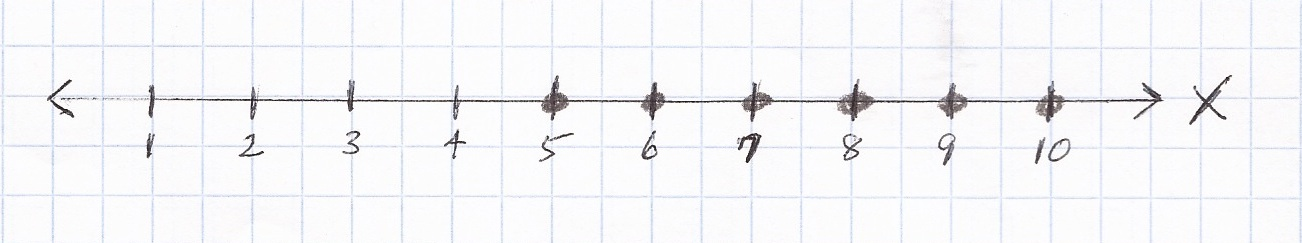Basics
An algebraic inequality can be plotted along a number line.

Sometimes, in order to plot an inequality, you will need to solve an equation.

Question

For x > 5,  plot points for whole numbers along the number line below.x > 5Because x > 5, plotting excludes 5, starts with 6, and proceeds higher from there.

Question

For x ≥ 5,  plot points for whole numbers along the number line below.x ≥ 5Because x ≥ 5, plotting includes 5 and proceeds higher from there.

Question

For x < 4, plot points for whole numbers along the number line below.x < 4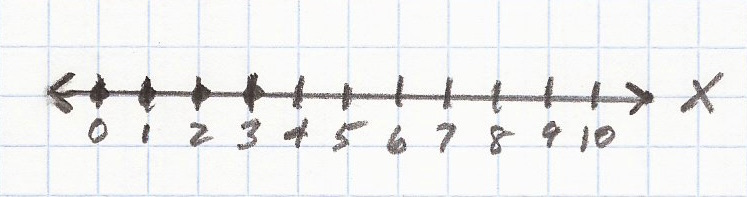Because x < 4, plotting excludes 4, starts with 3, and proceeds lower from there.

Question

For x ≤ 4, plot points for whole numbers along the number line below.x ≤ 4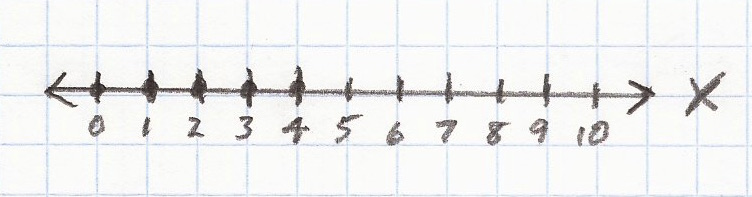Because x ≤ 4, plotting includes 4 and proceeds lower from there.

Question
A souvenir shop must generate $160.00 in order to break even. It spends$100.00 on supplies.  The shop will charge $12.00 for every souvenir x it sells. The inequality 12x + 100 ≥ 160 expresses the number of souvenirs the shop must sell in order to break even or make a profit. Plot the points for which the shop will break even or make a profit along the number line below.Answer x ≥ 5Answer Process See Equations. 12x + 100 ≥ 160 12x + 100 – 100 = 160 – 100 • 100 is subtracted from each side of = sign • subtraction is the opposite of addition 12x = 60 • 100 – 100 is the same as 0, so it disappears • 160 – 100 is the same as 60$\bf\displaystyle\frac{12x}{12}$$\bf\displaystyle\frac{60}{12}$ • each side of = sign is divided by 12 • division is the opposite of multiplication x = 5 •$\bf\displaystyle\frac{12x}{12}$ is the same as 1x which is the same as x$\bf\displaystyle\frac{60}{12}$ is the same as 5 • x has been isolated on the left side of = sign x ≥ 5 Because the inequality 12x + 100 ≥ 160 specifies greater than or equal to, points plotted along the number line include 5 and proceed higher from there. Calculator Click What You See Commentblinker clears screen 160 – 100 160-100 subtraction on right side of =6060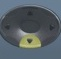12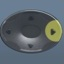$\bf\displaystyle\frac{60}{12}$ division on right side of =5 ###### Inequality Question A souvenir shop must generate greater than$160.00 in order to make a profit.  It spends $100.00 on supplies. The shop will charge$12.00 for every souvenir x it sells.

The inequality 12x + 100 > 160 expresses the number of souvenirs the shop must sell in order to make a profit.

Plot the points for which the shop will make a profit along the number line below.x > 5• The calculations are the same as in the previous queation.
• Because the inequality 12x + 100 > 160 specifies greater than, points plotted along the number line exclude 5, start with 6, and proceed higher from there.

Question
A souvenir shop must generate $163.00 in order to make a profit. It spends$100.00 on supplies.  The shop will charge $12.00 for every souvenir x it sells. The inequality 12x + 100 > 163 expresses the number of souvenirs the shop must sell in order to make a profit. Plot the points for which the shop will make a profit along the number line below.Answer x > 5.25, starting with 6Answer Process See Equations. 12x + 100 > 163 12x + 100 – 100 = 163 – 100 • 100 is subtracted from each side of = sign • subtraction is the opposite of addition 12x = 63 • 100 – 100 is the same as 0, so it disappears • 163 – 100 is the same as 63$\bf\displaystyle\frac{12x}{12}$$\bf\displaystyle\frac{63}{12}$ • each side of = sign is divided by 12 • division is the opposite of multiplication x = 5.25 •$\bf\displaystyle\frac{12x}{12}$ is the same as 1x which is the same as x$\bf\displaystyle\frac{63}{12}$ is the same as$\bf\displaystyle\frac{21}{4}$ which is the same as 5.25 after toggle • x has been isolated on the left side of = sign x > 5.25, starting with 6 • Because there is no such thing as 5.25 souvenirs and the inequality 12x + 100 > 163 specifies greater than, points plotted along the number line exclude 5, start with 6, and proceed higher from there. Calculator Click What You See Commentblinker clears screen 163 – 100 163-100 subtraction on right side of =636312$\bf\displaystyle\frac{63}{12}$ division on right side of =$\bf\displaystyle\frac{21}{4}$5.25 Toggle ###### Inequality Question A baseball card collector can spend up to$325.00 at a show.  The show charges a $25.00 entrance fee. The collector plans to spend$75.00 on each card x at the show.

The inequality 75x + 25 ≤ 325 expresses the number of baseball cards the collector can buy.  Plot the points representing all possible numbers of baseball cards the collector can buy along the number line below.x ≤ 4See Equations.

75x + 25 ≤ 325

75x + 25 – 25 = 325 – 25
• 25 is subtracted from each side of = sign
• subtraction is the opposite of addition

75x = 300
• 25 – 25 is the same as 0, so it disappears
• 325 – 25 is the same as 300$\bf\displaystyle\frac{75x}{75}$$\bf\displaystyle\frac{300}{75}$
• each side of = sign is divided by 75
• division is the opposite of multiplication

x = 4
•$\bf\displaystyle\frac{75x}{75}$ is the same as 1x which is the same as x$\bf\displaystyle\frac{300}{75}$ is the same as 4
• x has been isolated on the left side of = sign

x ≤ 4
• Because the inequality 75x + 25 ≤ 325 specifies less than or equal to, points plotted along the number line include 4 and proceed lower from there.  (Because the collector can choose to buy nothing, please note that point 0 should be plotted.)

Calculator Click What You See Commentblinker clears screen
325 – 25 325-25 subtraction on right side of =30030075$\bf\displaystyle\frac{300}{75}$ division on right side of =4
###### Inequality

Question
A baseball card collector must spend less than $325.00 at a show. The show charges a$25.00 entrance fee.  The collector plans to spend $75.00 on each card x at the show. The inequality 75x + 25 < 325 expresses the number of baseball cards the collector can buy. Plot the points representing the number of baseball cards the collector can buy along the number line below.Answer x < 4Answer Process • The calculations are the same as in the previous question. • Because the inequality 75x + 25 < 325 specifies less than, points plotted along the number line exclude 4, start with 3, and proceed lower from there. (Because the collector can choose to buy nothing, please note that point 0 should be plotted.) Question A baseball card collector must spend less than$325.00 at a show.  The show charges an $80.00 entrance fee. The collector plans to spend$75.00 on each card x at the show.

The inequality 70x + 80 < 325 expresses the number of baseball cards the collector can buy.

Plot the points representing the number of baseball cards the collector can buy along the number line below.x < 3.5, starting with 3See Equations.

70x + 80 < 325

70x + 80 – 80 = 325 – 80
• 80 is subtracted from each side of = sign
• subtraction is the opposite of addition

70x = 245
• 80 – 80 is the same as 0
• 325 – 80 is the same as 245$\bf\displaystyle\frac{70x}{70}$$\bf\displaystyle\frac{245}{70}$
• each side of = sign is divided by 70
• division is the opposite of multiplication

x = 3.5
•$\bf\displaystyle\frac{70x}{70}$ is the same as 1x which is the same as x$\bf\displaystyle\frac{245}{70}$ is the same as$\bf\displaystyle\frac{7}{2}$ which is the same as 3.5 after toggle
• x has been isolated on the left side of = sign

x < 3.5, starting with 3
• Because there is no such thing as 3.5 baseball cards and the inequality 70x + 80 < 325 specifies less than, points plotted along the number line exclude 4, start with 3, and proceed lower from there.  (Because the collector can choose to buy nothing, please note that point 0 should be plotted.)

Calculator Click What You See Commentblinker clears screen
325 – 80 325-80 subtraction on right side of =24524570$\bf\displaystyle\frac{245}{70}$ division on right side of =$\bf\displaystyle\frac{7}{2}$3.5 Toggle
###### Inequality

Practice – Questions

1.  For x < 8, plot points for whole numbers along the number line below.2.  For x ≤ 8, plot points for whole numbers along the number line below.3.  For x > 8, plot points for whole numbers along the number line below.4.  For x ≥ 8, plot points for whole numbers along the number line below.5.  A kiosk must generate $200.00 in order to break even. It spends$151.00 on supplies.  The kiosk will charge $7.00 for every item x it sells. The inequality 7x + 151 ≥ 200 expresses the number of items the kiosk must sell in order to break even or make a profit. Plot the points for which the kiosk will break even or make a profit along the number line below.6. A kiosk must sell more than$200.00 in order to make a profit.  It spends $151.00 on supplies. The kiosk will charge$7.00 for every item x it sells.

The inequality 7x + 151 > 200 expresses the number of items the kiosk must sell in order to make a profit.

Plot the points for which the kiosk will make a profit along the number line below.7.
A kiosk must sell more than $205.00 in order to make a profit. It spends$151.00 on supplies.  The kiosk will charge $7.00 for every item x it sells. The inequality 7x + 151 > 205 expresses the number of items the kiosk must sell in order to make a profit. Plot the points for which the kiosk will make a profit along the number line below.8. A tour group can spend up to$194.00 on a sightseeing trip.  The tour bus has a one-time reservation fee of $50.00. The tour bus charges$36.00 for each tourist x.

The inequality 36x + 50 ≤ 194 expresses the number of tourists the tour group can afford.

Plot the points representing the number of tourists the tour group can afford along the number line below.9.
A tour group must spend less than $194.00 on a sightseeing trip. The tour bus has a one-time reservation fee of$50.00.  The tour bus charges $36.00 for each tourist x. The inequality 36x + 50 < 194 expresses the number of tourists the tour group can afford. Plot the points representing the number of tourists the tour group can afford along the number line below.10. A tour group must spend less than$184.00 on a sightseeing trip.  The tour bus has a one-time reservation fee of $50.00. The tour bus charges$36.00 for each tourist x.

The inequality 36x + 50 < 184 expresses the number of tourists the tour group can afford.

Plot the points representing the number of tourists the tour group can afford along the number line below.1.  x < 8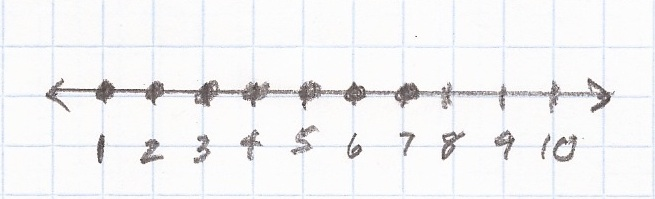2.  x ≤ 8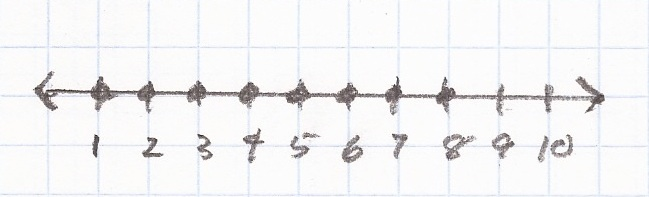3.  x > 85.  x ≥ 7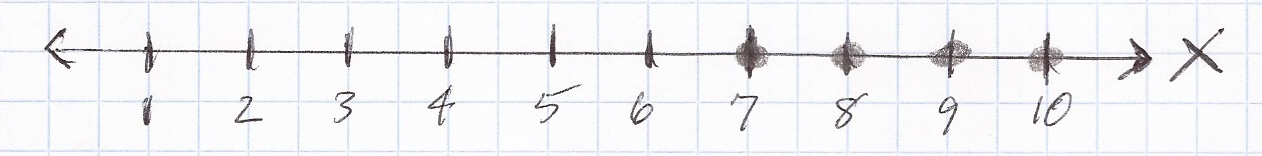6.  x > 7, starting with 8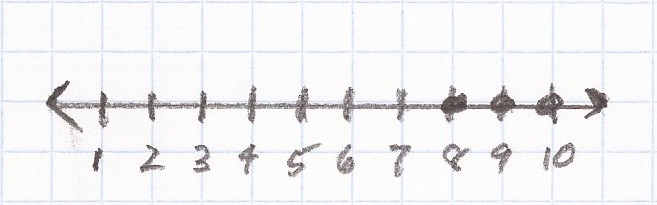7.  x > 7.71, starting with 88.  x ≤ 49.  x < 4, starting with 310.  x < 3.72, starting with 3